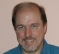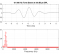## Generalized Cross Correlation method not producing desired resultsStarted by 7 years ago15 replieslatest reply 7 years ago1510 views

Hi,

I am trying to use generalized correlation method to estimate time delay between two sinusoid signals. Although without weighting cross-correlation method gives me the desired result, the ones with weights such as SCOT, PHAT, ROTH all produces a constant 0 delay. I used Matlab for coding. The code I have used for PHAT is as below:

FFTLength=400;
Rxx = xcorr(y1);
Ryy = xcorr(y2);
Rxy = xcorr(y1,y2);
Sxx = fft(Rxx,FFTLength);
Syy = fft(Ryy,FFTLength);
Sxy = fft(Rxy,FFTLength);

% Unfiltered Correlation (plain correlation)
W = ones(1,FFTLength);
% Apply the filter
R = Sxy.*W;
% Obtain the GCC
G = fftshift(real(ifft(R)),1);
figure(1);
plot(G);
title('Plain Time Cross Correlation');

% PHAT Filter
W = 1./abs(Sxy);
% Apply the filter
R = Sxy.*W;
% Obtain the GCC
G = fftshift(real(ifft(R)),1);
figure(1);
subplot(224);
plot(G);
title('Phat Kernel GCC');
[ - ]Generalized cross-correlation does not pertain to cross-correlating two sinusoids.  However, it's a good acid-test of the implementation which should not blow up at least due to division by zero.

If the sinusoidal frequencies are different, then the cross-correlation, generalized or not, is zero, so that's out.  You probably know this, but didn't specify.

When the frequencies are the same, you're just looking for the relative phase, and there are many ways to get that, including plain cross-correlation.

Generalized xcor additionally tries to "flatten" the spectrum, but the spectrum is an impulse and cannot be flattened!  A reasonable implementation should protect against divide-by-zero and boost the noise-floor up to the level of the sinusoid and give you cross-correlation of the noise floors.  A better implementation will have a dB threshold below which it does not try to flatten (say 60 dB below the peak) and give you back your sinusoid unchanged.  In other words, generalize xcor for a sinusoid should reduce to plain xcor.

[ - ]Yes so that means generalized xcorr should at least give the same results as a plain xcorr for two sinusoid signals. But in this case, it is not even giving that results correctly. Even if there is a delay, generalized xcorr is always returning 0.

[ - ]W = 1./abs(Sxy)

[ - ]Maybe change it to

W = 1./(1.0e-4 + abs(Sxy));
[ - ]Yes I even took care for that division by zero. Still no improvement :(

Here is the plot though its hard to understand so I will explain

For plain xcorr the peak is at 105 ( I took delay of 5 samples ) and for gcc the peak is at 100: which corresponds to 0 delay.

[ - ]I would also plot W, and limit its variation using the threshold I mentioned.

[ - ]Yes thats what I just did.

[ - ]Are your two sinusoidal signals just tones, or combined with other signal?

It would be helpful if you could make them available or Matlab code that generates examples of the signals you are working with.

[ - ]Initially I was working with 2 noisy signals but it didnt work for it. Then I took just two sinusoid signal for testing. and it didnt work for it as well. The code to generate the signals are:

x=1:100

y1=sin(2*pi*x/50);

y2=sin(2*pi*(x+5)/50);

The plot is given here: Plot

[ - ]Hi. Do you have a question for the people here?

[ - ]Yes,

So I was wondering what am I missing here. I know GCC is supposed to give better results than simple cross correlation method. What are the steps that I am doing wrong?

[ - ]Is this relevant?

[ - ]Yes, I have looked at this post before. The two replies relates to:
1. Using matlab function gccphat
2. Take a larger point fft, preferrably 4 times the length of the signal.

Unfortunately, none of them works for me. Even the matlab inbuilt function gccphat is not giving any expected results for two simple sinusiod delayed functions

[ - ]